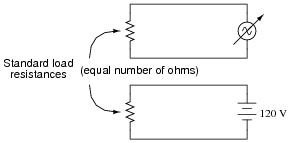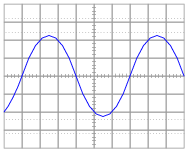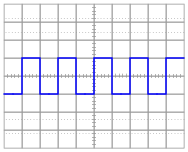# Peak, Average, and RMS Measurements

## AC Electric Circuits

• #### Question 1

How is it possible to assign a fixed value of voltage or current (such as “120 volts”) to an AC electrical quantity that is constantly changing, crossing 0 volts, and reversing polarity?

• #### Question 2

Suppose a DC power source with a voltage of 50 volts is connected to a 10 Ω load. How much power will this load dissipate?

Now suppose the same 10 Ω load is connected to a sinusoidal AC power source with a peak voltage of 50 volts. Will the load dissipate the same amount of power, more power, or less power? Explain your answer.

• #### Question 3

Suppose that a variable-voltage AC source is adjusted until it dissipates the exact same amount of power in a standard load resistance as a DC voltage source with an output of 120 volts:In this condition of equal power dissipation, how much voltage is the AC power supply outputting? Be as specific as you can in your answer.

• #### Question 4

Determine the RMS amplitude of this sinusoidal waveform, as displayed by an oscilloscope with a vertical sensitivity of 0.2 volts per division:• #### Question 5

Determine the RMS amplitude of this square-wave signal, as displayed by an oscilloscope with a vertical sensitivity of 0.5 volts per division:• #### Question 6

Suppose two voltmeters are connected to source of “mains” AC power in a residence, one meter is analog (D’Arsonval PMMC meter movement) while the other is true-RMS digital. They both register 117 volts while connected to this AC source.

Suddenly, a large electrical load is turned on somewhere in the system. This load both reduces the mains voltage and slightly distorts the shape of the waveform. The overall effect of this is average AC voltage has decreased by 4.5% from where it was, while RMS AC voltage has decreased by 6% from where it was. How much voltage does each voltmeter register now?

• #### Question 7

An electromechanical alternator (AC generator) and a DC-DC inverter both output the same RMS voltage, and deliver the same amount of electrical power to two identical loads:However, when measured by an analog voltmeter, the inverter’s output voltage is slightly greater than the alternator’s output voltage. Explain this discrepancy in measurements.

• #### Question 8

Is the deflection of an analog AC meter movement proportional to the peak, average, or RMS value of the waveform measured? Explain your answer.

• #### Question 9

In calculating the size of wire necessary to carry alternating current to a high-power load, which type of measurement is the best to use for current: peak, average, or RMS? Explain why.

• #### Question 10

In calculating the thickness of insulators for high-voltage AC power lines, which type of measurement is the best to use for voltage: peak, average, or RMS? Explain why.

### Related Content

Published under the terms and conditions of the Creative Commons Attribution License• F
Fabrizio_EL April 03, 2020

Hello, sorry but i doubt the asnwer to question #5, or at least i would ask for a better explanation.
For what i know, RMS amplitude of such a waveform is 1 x sqrt(0,5), while 0,5 seems to be average amplitude.

Like.
•RK37 April 06, 2020
The answer is correct. The signal—call it x(t)—is a square wave that transitions between 0.5 V and -0.5 V. To find the RMS value, we square x(t), then integrate it over a given period of time T, then divide by T, then take the square root. Let's say that x(t) has a period of 2 seconds. After squaring x(t), we have a signal that is a constant voltage of 0.25 V, because 0.5^2 = 0.25 and (-0.5)^2 = 0.25. If we integrate for T = 2 seconds, the result is 0.25 * 2 = 0.5. Dividing by T, we have 0.5/2 = 0.25, and taking the square root of 0.25 yields 0.5. This works for any number that you choose for T. The important concept is the following: If you square a signal like the one shown in the diagram, you end up with a constant value. The average value of a constant value is the same constant value, and thus when you take the square root, you "undo" the square operation and return to the original amplitude.
Like.
• F
Fabrizio_EL April 14, 2020
Many thanks, actually my (huge) mistake was to consider that waveform from 0 to 1, since i didn't notice the "0" line through it. So i used the formula Vrms = sqrt( d% * Vhigh^2 + (1-d%) * Vlow^2 ), with d = 0,5, Vhigh = 1, Vlow = 0. Using instead this formula with the correct values Vhigh = 0,5 and Vlow = -0,5 leads to your same result. And now that you explained with simple integral calculus i can see the equivalence (mathwise) between the two methods. Thank you again for being so clear.
Like.